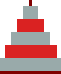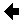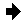## The "mysterious" TH algorithm explained &Configuration of all disks from the move countM. Kolar
March 4; revised March 10, 2000

### 1. The "mysterious" TH algorithm explained

Here I show that the "mysterious" TH algorithm is a variation of the cyclic algorithm (see here Schoute (ref ); my Algorithm no. 4), which explicitly uses the move count k (denoted as x in hBits.c).

G.C. Rhoads labels the pegs 0, 1 and 2. Peg 0 is the start peg, and the final peg is either 1 (for even number of disks n) or 2 (for odd n). This has the advantage that the initial common moves are the same for all n, e.g., the first move is always from peg 0 to peg 2, etc. And the cyclic order is the same for all n: 0 -> 2 -> 1 -> 0. This cyclic order can be written e.g. as a series of even numbers modulo 3: (2i)%3, i=0,1,2,...

Disks have the usual labels from 1 (the smallest) to n (the largest).

Odd disks are always moved according to the cyclic order, even disks in the opposite order. The cyclic algorithm consists in repeating the two following steps (an odd numbered move followed by an even numbered one):

1. Move disk 1 to the next peg in the cyclic order;
2. If all disks are on the same peg, exit. Else move a disk between the two pegs that are not topped by disk 1 - naturally, the smaller of the two disks on top of these two pegs is moved.

Thus disk 1 is moved in all odd (numbered) moves (k=1,3,5,...). One starts from peg 0. In move 1 (k=1), disk 1 is moved from peg 0 to peg 2, in move 3 (k=3) from peg 2 to peg 1, in move 5 (k=5) from peg 1 to peg 0, etc., always in the cyclic order. In the above modulo 3 representation of the cyclic order this can be written as follows: disk 1 is moved in each odd move k from peg (k-1)%3 to peg (k+1)%3.

If the disk moved in step 2 is also odd, it must be different from disk 1, and so be moved from peg that follows in the cyclic order the final peg of the move of disk 1 of step 1. If k is the even move number for step 2, the final peg for disk 1 in the previous odd move k-1 was (k-1+1)%3=k%3, and thus the step 2 move is from peg (k+2)%3 to peg (k+4)%3.

However, (k+2)%3=(k-1)%3 and (k+4)%3=(k+1)%3, and thus if an odd disk is moved in move k (odd or even), the move can always be written as a move from peg (k-1)%3 to peg (k+1)%3.

If the disk moved in step 2 is even, it is moved between the same two pegs, but in the direction opposite to the cyclic order. That is, from peg (k+4)%3 to peg (k+2)%3. Because in this case k>1, the starting peg of this move can also be written as (k-2)%3.

Now let us denote by j the number labeling the disk that is moved in move k. By induction one has (22i+1)%3 = 2 and (22i)%3 = 1 for arbitrary nonnegative i. For odd j=2i-1 we then have (k-1)%3 = (k-2j-1)%3, and for even j=2i+2 we have (k-2)%3 = (k-2j-1)%3; i>=0. Similarly for the final peg numbers. Thus for arbitrary k and j, the move can always be written as from peg (k-2j-1)%3 to peg (k+2j-1)%3.

Next, we will use the following property of binary representation of a nonnegative integer k: if k is terminated with Z consecutive zeros preceded by a one, the binary representation of k-1 ends with Z consecutive 1's preceded by a 0. Therefore, k|(k-1) = k+2Z-1 and k&(k-1) = k-2Z, where | and & are bitwise OR and bitwise AND operations of the C language.

Finally, as mentioned before, for move k, j=Z+1. Putting all these relations together gives that in move k, disk j=Z+1 is moved from peg (k&(k-1))%3 to peg (k|(k-1)+1)%3, which is exactly what is used in hBits.c.

(Note: For all k, one also has k^(k-1) = 2j+1-1, where ^ is C's bitwise exclusive OR.)

### 2. Configuration of all disks can also be obtained from the bit representation of the move count k

In this Section, the disks are numbered as above, but the pegs have the usual numbering 1, 2 and 3, and the start peg is 1 and the final peg is always 3. The empty pegs (bases of the pegs) are also treated as disks with numbers n+1, n+2 and n+3.

The disk configuration is obtained from the following Theorem proved in :

After 0 <= k <= 2n-1 moves,

1. Each disk is on a disk or empty peg of opposite parity;

2. Exactly one of the top labels (disk or empty peg) is even;

3. If jn+1jn...j1 is the binary representation of k, then for m=n,n-1,...,1, disk m is on disk (or empty peg) m+1 if and only if jm=jm+1 (since jn+1=0 for 0 <= k <= 2n-1, the case where m=n means that disk n in on peg 1 iff jn=0).

A possible implementation of this Theorem can be find in moveConf.c, which is now also contained in the revised TH C zip archive. Its essential part is as follows:

 ``` /* Using T.R. Walsh' Theorem: p[m] - peg on which the m-th disk is placed b - tests bits of k jm1, jm - values of 2 consecutive bits of k */ p= (int *)malloc((n+4) * sizeof(int)); p[n+1]= 1; /* base of peg 1, etc. */ p[n+2]= 2; p[n+3]= 3; b= 1<0; m--) { jm1= jm>>1; b >>= 1; jm= k&b; if(jm == jm1) p[m]= p[m+1]; else { for(i=m+2; i0;m--) if(p[m]==1) PR" %d",m); PR"\n2|"); for(m=n;m>0;m--) if(p[m]==2) PR" %d",m); PR"\n3|"); for(m=n;m>0;m--) if(p[m]==3) PR" %d",m); PR"\n"); ```
The core of moveConf.c

It looks quite complicated. Would somebody be able to simplify this code?

The above Theorem can also be used in the opposite direction: given a configuration of disks, one can determine the corresponding move count k, if the configuration occurs in the minimal TH solution, or conclude that it is an illegal configuration. For further details, see Ref. .

Acknowledgment: Thanks to Paul Stockmeyer for making me aware of Ref. 1, and Romek Zylla for e-mailing me a copy of it.

References
  T.R. Walsh, The Towers of Hanoi revisited: moving the rings by counting the moves, Information Processing Letters, 1982, Vol.15, 64-67. Netherlands.